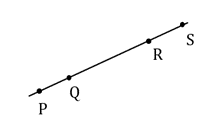Mathematics
Easy

Question

# What is the 3rd reason?Given: Q is between P and R, R is between Q and S, PR = QS.Prove: PQ = RSProof:Statements Reason 1. Q is between P and R. 1. Given 2. PQ + QR = PR 2. ________ 3. R is between Q and S. 3. _________ 4. _______ 4. Seg. Add. Post 5. PR = QS 5. _______ 6. PQ + QR = QR + RS 6. ________ 7. PQ + QR – QR = QR + RS - QR 7. ________ 8. ____________ 8. Substitution

## definition of congruencetransitive propertydefinition of midpointgivenHint:

## The correct answer is: given

### Here we have to find the reason of step 3.Firstly , we must consider that PQRS as a straight line, and P,Q, R and S are point on it one after another.Here given is PR = QS 1 . Q is between P and R ( given)2. PQ + QR = PR (Segment addition Postulate)3. R is between Q and S ( given )4. QR + RS =QS ( Segment addition Postulate )5. PR = QS ( given)6. PQ +QR = QR + RS ( substitution )7. PQ + QR – QR = QR + RS – QR (subtraction property , subtract from QR)8. PQ = RS ( substitution )Therefore, The reason of step3 is given.The correct answer is given.

In this question , Most of steps are used as segment addition property to prove that PQ = RS . In substation property , If A =B and A = C then we can write A = C.

### Related Questions to study#### With Turito Foundation.#### Get an Expert Advice From Turito.